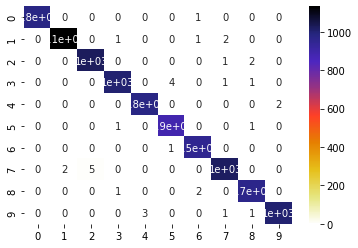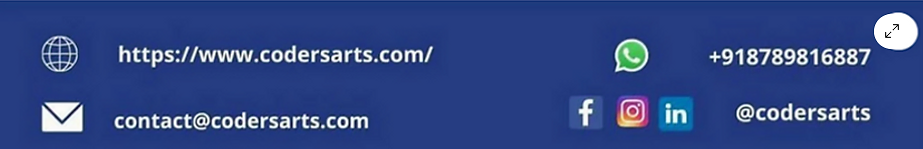top of page
Search

# Beginners' Guide to Classification using MNIST Digit Dataset

Updated: Jan 5

INTRODUCTION

In deep learning, we use classification algorithms to tackle the problem of identifying objects. One such problem is to train a deep learning model to identify the digits present in the images. To do this, we will use the MNIST dataset that consists of the images of digits. First, we will train the model using the MNIST dataset, and then we will test the model on new instances of images.

While doing these, you will learn various concepts like normalizing and reshaping the images, data augmentation to prevent the overfitting, one-hot encoding to encode the labels into suitable form, and evaluation of the model using a learning curve graph.

IMPORT THE ESSENTIAL LIBRARIES

First we will import the libraries to work with.

```import warnings
warnings.filterwarnings('ignore')

import numpy as np
import pandas as pd
import matplotlib.pyplot as plt.
import seaborn as sns

from sklearn.metrics import confusion_matrix

from keras.utils.np_utils import to_categorical
from keras.models import Sequential
from keras.layers import Dense, Dropout, Flatten, Conv2D, MaxPool2D
from keras.optimizers import RMSprop
from keras.preprocessing.image import ImageDataGenerator
from keras.callbacks import ReduceLROnPlateau```

IMPORT THE DATASET

```from keras.datasets import mnist

(X_train, y_train), (X_test, y_test) = mnist.load_data()```

SHAPE OF THE DATASET

```print("X_train Shape", X_train.shape)
print("X_test Shape", X_test.shape)
print("y_train Shape", X_train.shape)
print("y_train Shape", X_train.shape)
print("X_train datatype", X_train.dtype)```

OUTPUT

```X_train Shape (60000, 28, 28)
X_test Shape (10000, 28, 28)
y_train Shape (60000, 28, 28)
y_train Shape (60000, 28, 28)
X_train datatype uint8```

RESHAPING

Expanding one more dimension for color channel gray

```X_train = X_train.reshape(X_train.shape, 28, 28,1)
print("X_train Shape", X_train.shape)

X_test = X_test.reshape(X_test.shape, 28, 28,1)
print("X_test Shape", X_test.shape)```

OUTPUT

```X_train Shape (60000, 28, 28, 1)
X_test Shape (10000, 28, 28, 1)```

NORMALIZATION

```# Normalize the data
X_train = X_train / 255.0
X_test = X_test / 255.0```

CHECKING DATA IMBALANCE

```sns.countplot(y_train)
plt.show()```

OUTPUTPERFORMING ONE-HOT ENCODING ON TARGET SET

```y_train = to_categorical(y_train, num_classes = 10)
y_test = to_categorical(y_test, num_classes = 10)```

DEFINING THE DEEP LEARNING MODEL ARCHITECTURE

```def define_model():
model = Sequential()

activation ='relu', input_shape = (28,28,1)))
activation ='relu'))

activation ='relu'))
activation ='relu'))

return model

```

LEARNING RATE REDUCTION CONFIGURATION

```lr_reduction_config = ReduceLROnPlateau(monitor = 'val_accuracy',
patience = 3,
verbose = 1,
factor = 0.5,
min_lr = 0.00001)```

PERFORMING DATA AUGMENTATION

```datagen = ImageDataGenerator(
featurewise_center=False,
rotation_range=10,
zoom_range = 0.1,
width_shift_range=0.1,
height_shift_range=0.1,
horizontal_flip=False,
vertical_flip=False)

datagen.fit(X_train)```

CREATING A MODEL AND TRAINING THE MODEL

```# Fit the model
model = define_model()

# Compile the model
model.compile(optimizer = 'adam' , loss = "categorical_crossentropy", metrics=["accuracy"])

history = model.fit_generator(datagen.flow(X_train,y_train, batch_size = 64),
epochs = 30, validation_data = (X_test,y_test),
verbose = 2, steps_per_epoch=X_train.shape // batch_size
, callbacks=[lr_reduction_config])```

```Epoch 1/30 937/937 - 21s - loss: 0.3079 - accuracy: 0.9025 - val_loss: 0.0383 - val_accuracy: 0.9878 - lr: 0.0010 - 21s/epoch - 22ms/step
..............................
..............................
937/937 - 18s - loss: 0.0157 - accuracy: 0.9952 - val_loss: 0.0101 - val_accuracy: 0.9966 - lr: 1.2500e-04 - 18s/epoch - 19ms/step```

```# plot accuracy and loss
def plotgraph_accuracy(epochs, acc, val_acc):
# Plot training & validation accuracy values
plt.plot(epochs, acc, 'b')
plt.plot(epochs, val_acc, 'r')
plt.title('Model accuracy')
plt.ylabel('Accuracy')
plt.xlabel('Epoch')
plt.legend(['Train', 'Val'], loc='upper left')
plt.show()```

```# plot accuracy and loss
def plotgraph_loss(epochs, loss, val_loss):
# Plot training & validation accuracy values
plt.plot(epochs, loss, 'b')
plt.plot(epochs, val_loss, 'r')
plt.title('Model loss')
plt.ylabel('Loss')
plt.xlabel('Epoch')
plt.legend(['Train', 'Val'], loc='upper left')
plt.show()```

```# Get the accuracy, loss, and other information
acc = history.history['accuracy']
val_acc = history.history['val_accuracy']
loss = history.history['loss']
val_loss = history.history['val_loss']
epochs = range(1,len(loss)+1)```
```# Plot the ACCURACY VS EPOCH
plotgraph_accuracy(epochs, acc, val_acc)``````# Plot the LOSS VS EPOCH
plotgraph_loss(epochs, loss, val_loss)````y_pred = model.predict(X_test)`
```predictions = []
for i in y_pred:
predictions.append(np.argmax(i))```
```ground_truth = []
for i in y_test:
ground_truth.append(np.argmax(i))```
```cm = confusion_matrix(predictions, ground_truth)
cmap_value = 'CMRmap_r'
sns.heatmap(cm, annot = True, cmap = cmap_value)
plt.show()```If you are looking for help in Django project contact us contact@codersarts.combottom of page